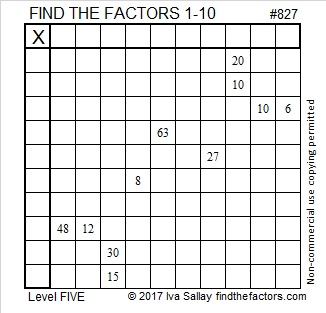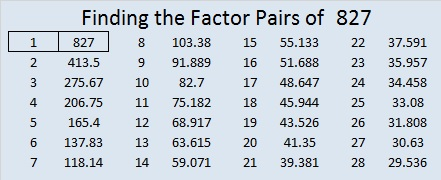# 827 and Level 5

827 is one of the prime numbers in the fourth prime decade, (821, 823, 827, 829).

827 = 103 + 107+ 109 + 113+ 127 + 131 + 137, that’s the sum of 7 consecutive prime numbers.

Here’s today’s puzzle:Print the puzzles or type the solution on this excel file: 10-factors-822-828

• 827 is a prime number.
• Prime factorization: 827 is prime.
• The exponent of prime number 827 is 1. Adding 1 to that exponent we get (1 + 1) = 2. Therefore 827 has exactly 2 factors.
• Factors of 827: 1, 827
• Factor pairs: 827 = 1 × 827
• 827 has no square factors that allow its square root to be simplified. √827 ≈ 28.7576How do we know that 827 is a prime number? If 827 were not a prime number, then it would be divisible by at least one prime number less than or equal to √827 ≈ 28.8. Since 827 cannot be divided evenly by 2, 3, 5, 7, 11, 13, 17, 19, or 23, we know that 827 is a prime number.This site uses Akismet to reduce spam. Learn how your comment data is processed.